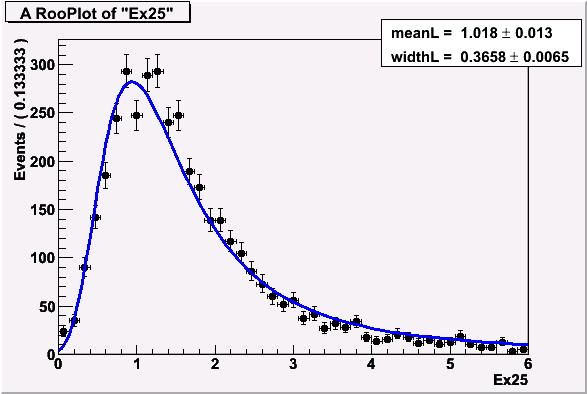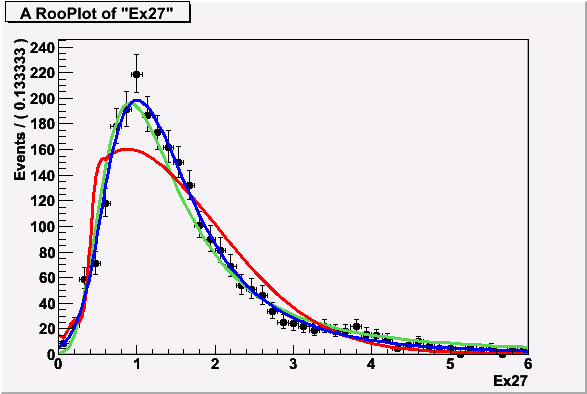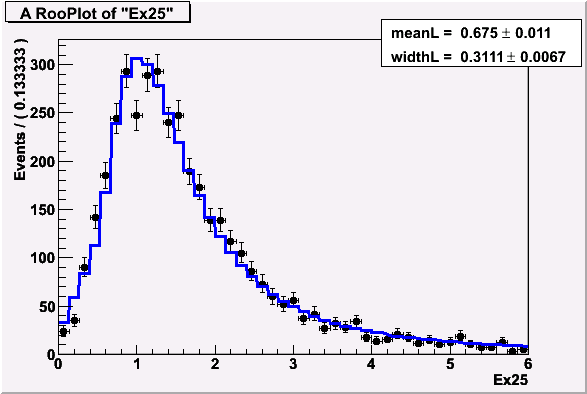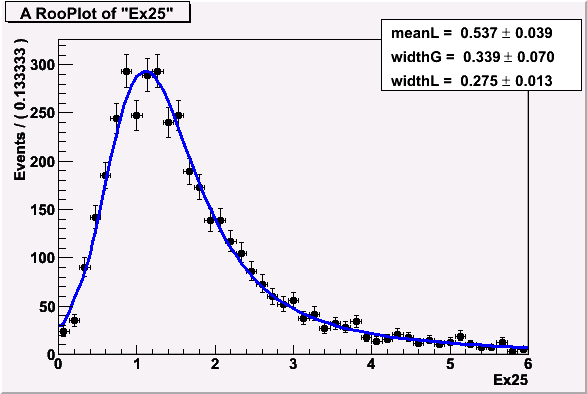# RooFFTConvPdf

I fitted histogram with Landau distribution and got parameters mean 1.018 and width 0.3658. (fitLandau.gif)

Then I decided fit histogram more correct and used RooFFTConvPdf for convolution RooLandau with RooGaussian. I fixed mean of Gauss as 0, it fitted better and got three other parameters meanLandau = 0.537, widthLandau = 0.275, sigma = 0.339. (fitConv.gif) Is it correct that mean of Landau is so far from real position of peak?

Then I made convolution with very narrow Gauss. I fixed mean of Gauss 0 and width of Gauss 0.01. (fitConvDeltaGauss.gif) I got meanLandau = 0.675. Why convolution with narrow Gauss gives results so differ from usual Landau?

Also RooFFTConvPdf doesn’t fit histogram also. (convDidnotFit.gif) Green line is RooLandau, blue is RooBukinPdf and red is RooFFTConvPdf (convolution Landau with Gauss).other attachments